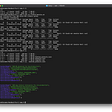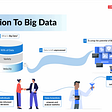# Data Visualisation

## 1. Plot a simple bar graph with two arrays.

`plt.bar([0,1,2,3],[12,23,21,8])`
`plt.bar([0,1,2],[34,46,23]) #Production in 2018plt.bar([0,1,2],[23,45,43]) #Production in 2019`
`pos_x=np.array([0,1,2])plt.bar(pos_x,[34,46,23]) #Production in 2018plt.bar(pos_x+0.25,[23,45,43]) #Production in 2019`
`pos_x=np.array([0,1,2])plt.bar(pos_x,[34,46,23], width=0.5) #Production in 2018plt.bar(pos_x+0.5,[23,45,43], width=0.5) #Production in 2019`
`pos_x=np.array([0,1,2])*2plt.bar(pos_x,[34,46,23], width=0.5) #Production in 2018plt.bar(pos_x+0.5,[23,45,43], width=0.5) #Production in 2019`

## 2. Give Labels

`pos_x=np.array([0,1,2])*2plt.bar(pos_x,[34,46,23], width=0.5, label=”2018") #Production in 2018plt.bar(pos_x+0.5,[23,45,43], width=0.5, label=”2019") #Production in 2019plt.legend()`

## 3. Put labels on x-axis

`pos_x=np.array([0,1,2])*2plt.bar(pos_x,[34,46,23], width=0.5, label=”2018", tick_label=[“Jan”, “Feb”, “Mar”]) #Production in 2018plt.bar(pos_x+0.5,[23,45,43], width=0.5, label=”2019") #Production in 2019plt.legend()`

## 4. Give a title to the plot and both the axes

`pos_x=np.array([0,1,2])*2plt.bar(pos_x,[34,46,23], width=0.5, label=”2018", tick_label=[“Jan”, “Feb”, “Mar”]) #Production in 2018plt.bar(pos_x+0.5,[23,45,43], width=0.5, label=”2019") #Production in 2019plt.title(“Wheat Production”)plt.xlabel(“Months”)plt.ylabel(“Production in Kgs”)plt.legend()`
`plt.style.use(“dark_background”)`

## 5. Change the color of bars

`plt.style.use(“dark_background”)pos_x=np.array([0,1,2])*2plt.bar(pos_x,[34,46,23], width=0.5, label=”2018", tick_label=[“Jan”, “Feb”, “Mar”], color=”blue”) #Production in 2018plt.bar(pos_x+0.5,[23,45,43], width=0.5, label=”2019", color=”orange”) #Production in 2019plt.title(“Wheat Production”)plt.xlabel(“Months”)plt.ylabel(“Production in Kgs”)plt.legend()`

## More from Shatakshi Singh

Love podcasts or audiobooks? Learn on the go with our new app.

## Databricks: Setting up Our Spark Dataframe for Linear Regression## Doctors keep getting abandoned. What can we do for them?## Intelligent Logistics## Moving my data science blog to medium## How to Get All Stocks from the S&P500 in Python## Human Resource Analytics using Machine Learning## 9 Feb ’21: Responsible Tech Bulletin## Making Good Metrics## Simplify the license consumption metrics retrieval of your Cloud Pak cluster## Are you afraid of Deepnude Algorithms? Tattoos might help.## Introduction To Big Data | Types & It’s Characteristics## Benchmark Design